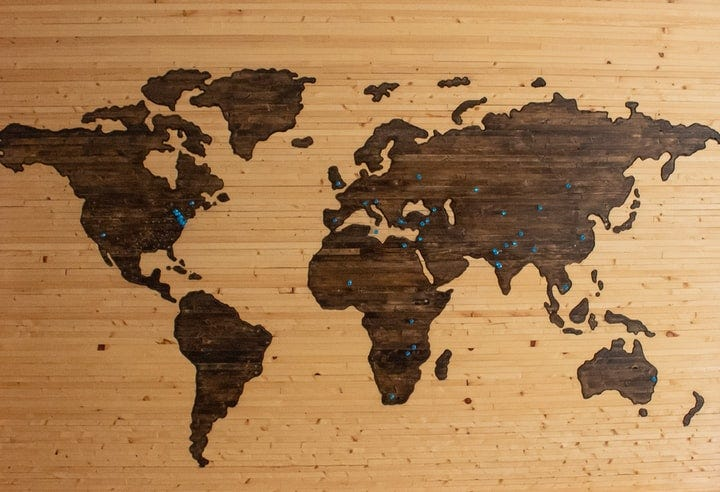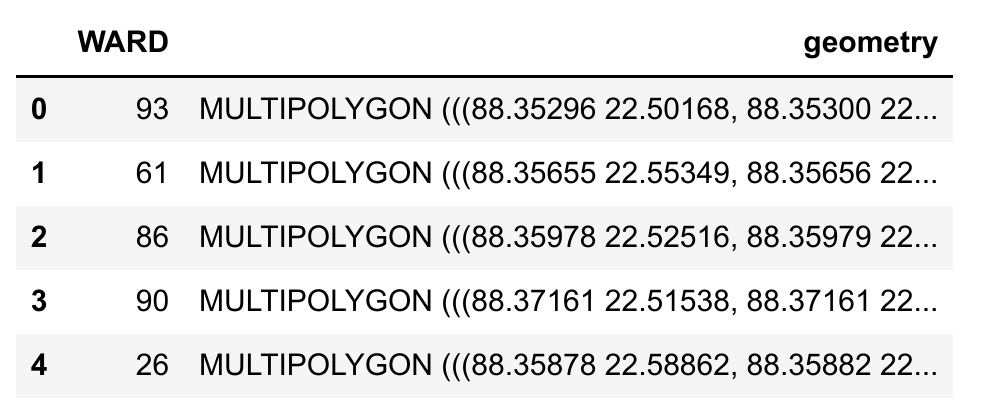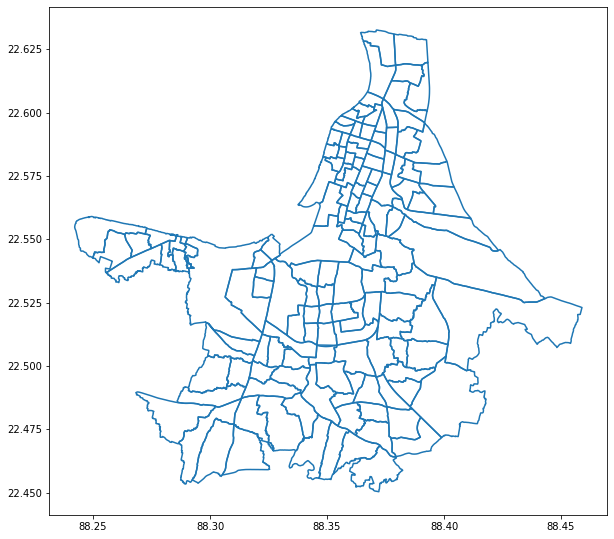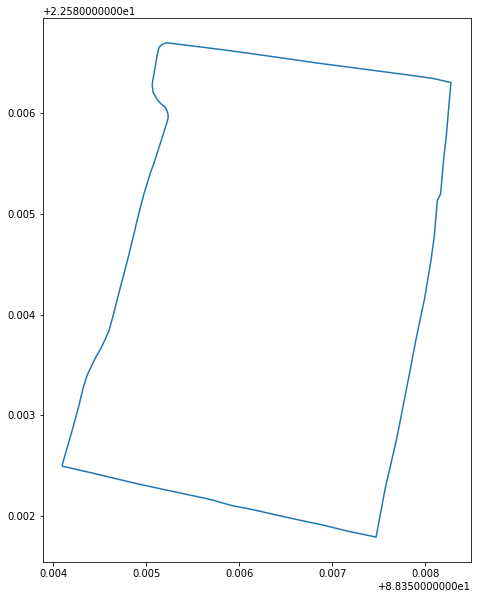# Visualization of GeoJSON Map using GeoPandasPhoto by Brett Zeck on Unsplash

In this tutorial, we will learn how to visualize a GeoJSON map using Python’s GeoPandas library. In this tutorial, we will use GeoJSON map data which are available on this link. This GeoJSON file corresponds to the Kolkata municipal corporation map data with ward-specific information.

## Import the GeoPandas package

We can import the GeoPandas package using the following command:

import geopandas as gpd

If you don’t have the GeoPandas package installed on your system then you can use the following pip command:

pip install geopandas

## Read the GeoJSON file

Now, we are ready to read the map data from a GeoJSON file. This can be done using the following command:

This data contains information on the wards of the Kolkata Municipal Corporation in West Bengal, India. The GeoJSON file can be downloaded from this link.

Disclaimer: In this tutorial, the data set is used for educational purposes only. The author of this tutorial does not verify the authenticity and the correctness of the information in the GeoJSON file.

## Plot the GeoJSON file

It is very simple to plot the GeoJSON file using GeoPandas. The command is shown below:

df.plot()

The above command will show the following figure:

We can also pass a few parameters in the plot() function to change the view of the figure as follows:

df.plot(figsize=(10,10), edgecolor=‘purple’, facecolor=‘green’);

This will show the following figure:

## Analysis of the GeoJSOn file

We can also analyze the GeoJSON file. We can determine the shape of the input data as follows:

df.shape

The output will be a tuple as shown below:

(141, 2)

Therefore, the input file kolkata.geojson has 141 rows and 2 columns (i.e., attributes). It also means that Kolkata municipality has 141 wards according to the input data file.

We can also view the first five rows of the input data as follows:

The output is shown below:Fig. First five rows of kolkata.geojson file

We can see that the attribute names are “WARD” and “geometry”.

Subscribe to the YouTube channel of Dr. Soumen Atta, Ph.D.

## More plot options

It may happen that we want to plot the boundaries of the wards only. This can be easily done using the following command:

df.boundary.plot(figsize=(10,10));

The output is shown below:Fig. Boundaries of all the wards of Kolkata

If we want to plot the boundary of a particular ward, say ward number 23, in Kolkata municipality then we can use the following commands:

df_23 = df.loc[df[‘WARD’] == ‘23’]
df_23.boundary.plot(figsize=(10,10));

Alternatively, we can also use the following commands:

df_23 = df[df.WARD==‘23’]
df_23.boundary.plot(figsize=(10,10));

The output figure is shown below:Fig. Boundary of ward 23 in Kolkata

This is the end of this tutorial where we have learned how to read a GeoJSON file using Python’s GeoPandas library and then we have learned how to plot the GeoJSON file in different ways.

--

--## Dr. Soumen Atta, Ph.D.

Postdoctoral Researcher at Laboratoire des Sciences du Numérique de Nantes (LS2N), Université de Nantes, IMT Atlantique, Nantes, France.